# Learn C Language-Tutorial 4: Decision Control Instructions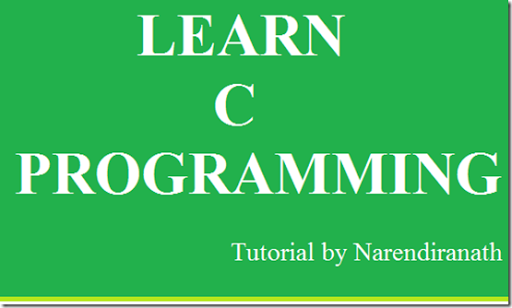In this tutorial we will learn about the Decision Control Instructions which makes a basics for any applications. In real time there are several conditions applied to complete a task.
In C programming we can implement the conditions by using these Decision Control Instructions.
They are
1.The if statement
2.The if-else statement
3.The conditional operator
Let we look in detail about these…
The if statement:
The if  is a keyword in  C language to implement the decision control instructions.
The general form of if statement is
if(this condition is true)
execute this statement;
The condition that we have to apply is written within the parenthesis.
Let us see an example
#include<stdio.h>
#include<conio.h>
void main()
{
int a;
clrscr();
printf(“\n Enter the number less than 10”);
scanf(“%d”,&a);
if(a<10)
printf(“you have entered corresct number”);
return 0;
}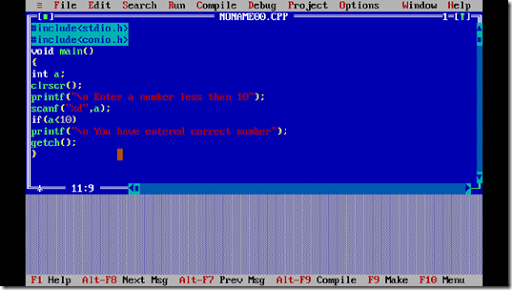Compile it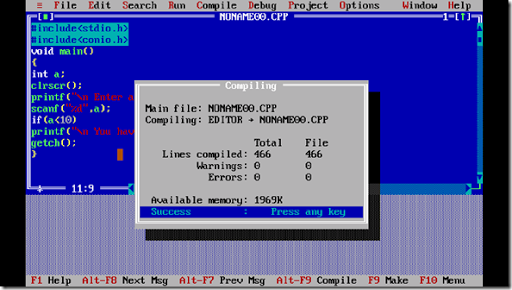Run it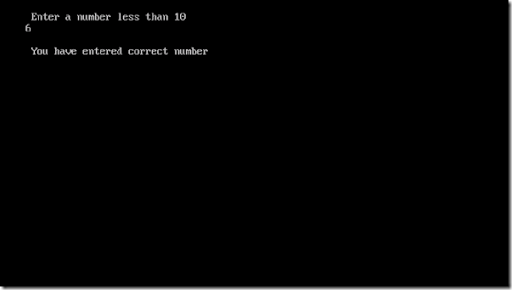if you enter the number greater than 10 then the output screen will be returned to the programming page.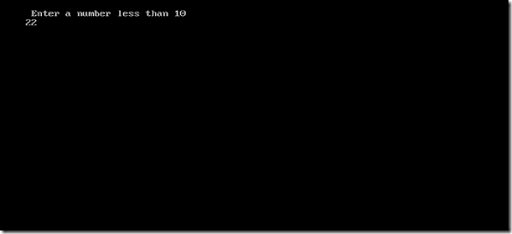In the above program if the wrong input is given the system will not show anything to the user it just change the output screen to the original screen.There is no notification that the users input is wrong thus an another conditional statement is used it is the if-else statement.
The if-else statement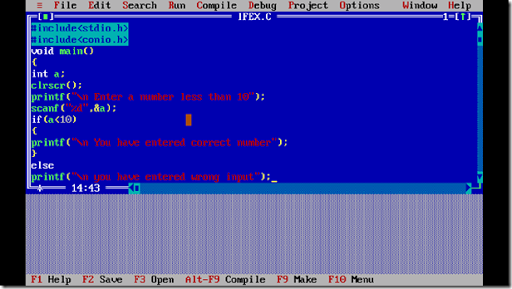#include<stdio.h>
#include<conio.h>
void main()
{
int a;
clrscr();
printf(“\n Enter the number less than 10“);
scanf(“%d”,&a);
if(a<10)
{
printf(“\n You have entered correct number”);
}
else
printf(“\n you have entered wrong number”);
getch();
}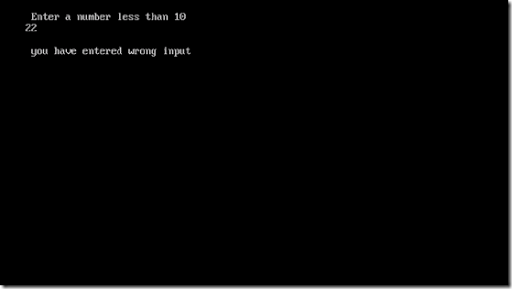Thus we are getting the output
Forms of IF
the if statement take the following forms
1. if(condition)
do this;
2.if(condition)
{
do this;
and this;
}
3.if(conditon)
do this;
else
do this;
4.if(condition)
{
do this;
and this;
}
else
{
do this;
and this;
}
5.if(condition)
do thus;
else
{
if(condition)
do this;
else
{
do this;
and this;
}
}
if(condition)
{
if(condition)
do this;
else
{
do this;
and this;
}
}
else
do this;

Use of Logical Operator

C language supports 3 logical operators 'AND' operator '&&','OR'operator ' ||' , and 'NOT' ! operators.
&& - this operator is to check the conditions and it will expect that every conditions associated with must be true otherwise it will not be executed.

||- The OR operator will be satisfied with any of its associated condition is true.

!- The NOT operator will perform not equal to operation.

Let us see an example to understand the above operators.

#include<stdio.h>
#include<conio.h>
void main()
{
int m1,m2,m3,m4,m5,tot,per;
printf("\n Enter the marks of 5 subjects");
scanf("%d%d%d%d%d",&m1,&m2,&m3,&m4,&m5);
tot=m1+m2+m3+m4+m5;
per=tot/5;
if(m1>=35&&m2>=35&&m3>=35m4>=35&&m5>=35)
{printf("\n pass");}
else
printf("\n Fail");
if(m1>85||m2>85||m3>85||m4>85||m5>85)
{
printf("\n eligible for distinction")
}
else
printf("\n Not elligible");
if(per!>85)
{
printf("\n not a rank holder");
}
else
printf("\nCongradulations... You are a  Rank Holder");
getch();
}

Program Explanation:

In the above program pass will be displayed only if all the marks are greater than or equal to 35.

The eligible for distinction if any one of the mark is greater than 85.

The Rank holder is decided if the percentage 'per' is not greater than 85.

I thing you have understood the Logical operator...

The Conditional Operator

The Conditional operators ? and : sometimes called as ternary operators since they take three arguments.

Their general form is

expression 1? expression 2:expression 3

that is  "if  expression 1 is true then the value returned will be expression 2 otherwise the value returned will be expression 3"

Let us see an example:

#include<stdio.h>
#include<conio.h>
void main()
{
int a,b;
clrscr();
printf("\n Enter the number");
scanf("%d",&a);
b=(a>10?5:6);
printf("\n the result is %d",b);
getch();
}

Thus in this program if the value of a is greater than 10 then the value of b is 5 otherwise the value is 6.

That's all guys we have done learning this tutorial of Decision Control Structure

read it , any doubt comment below if any doubt , share it ......